# 1-D Haar Wavelets¶

Important: Please read the installation page for details about how to install the toolboxes. $\newcommand{\dotp}{\langle #1, #2 \rangle}$ $\newcommand{\enscond}{\lbrace #1, #2 \rbrace}$ $\newcommand{\pd}{ \frac{ \partial #1}{\partial #2} }$ $\newcommand{\umin}{\underset{#1}{\min}\;}$ $\newcommand{\umax}{\underset{#1}{\max}\;}$ $\newcommand{\umin}{\underset{#1}{\min}\;}$ $\newcommand{\uargmin}{\underset{#1}{argmin}\;}$ $\newcommand{\norm}{\|#1\|}$ $\newcommand{\abs}{\left|#1\right|}$ $\newcommand{\choice}{ \left\{ \begin{array}{l} #1 \end{array} \right. }$ $\newcommand{\pa}{\left(#1\right)}$ $\newcommand{\diag}{{diag}\left( #1 \right)}$ $\newcommand{\qandq}{\quad\text{and}\quad}$ $\newcommand{\qwhereq}{\quad\text{where}\quad}$ $\newcommand{\qifq}{ \quad \text{if} \quad }$ $\newcommand{\qarrq}{ \quad \Longrightarrow \quad }$ $\newcommand{\ZZ}{\mathbb{Z}}$ $\newcommand{\CC}{\mathbb{C}}$ $\newcommand{\RR}{\mathbb{R}}$ $\newcommand{\EE}{\mathbb{E}}$ $\newcommand{\Zz}{\mathcal{Z}}$ $\newcommand{\Ww}{\mathcal{W}}$ $\newcommand{\Vv}{\mathcal{V}}$ $\newcommand{\Nn}{\mathcal{N}}$ $\newcommand{\NN}{\mathcal{N}}$ $\newcommand{\Hh}{\mathcal{H}}$ $\newcommand{\Bb}{\mathcal{B}}$ $\newcommand{\Ee}{\mathcal{E}}$ $\newcommand{\Cc}{\mathcal{C}}$ $\newcommand{\Gg}{\mathcal{G}}$ $\newcommand{\Ss}{\mathcal{S}}$ $\newcommand{\Pp}{\mathcal{P}}$ $\newcommand{\Ff}{\mathcal{F}}$ $\newcommand{\Xx}{\mathcal{X}}$ $\newcommand{\Mm}{\mathcal{M}}$ $\newcommand{\Ii}{\mathcal{I}}$ $\newcommand{\Dd}{\mathcal{D}}$ $\newcommand{\Ll}{\mathcal{L}}$ $\newcommand{\Tt}{\mathcal{T}}$ $\newcommand{\si}{\sigma}$ $\newcommand{\al}{\alpha}$ $\newcommand{\la}{\lambda}$ $\newcommand{\ga}{\gamma}$ $\newcommand{\Ga}{\Gamma}$ $\newcommand{\La}{\Lambda}$ $\newcommand{\si}{\sigma}$ $\newcommand{\Si}{\Sigma}$ $\newcommand{\be}{\beta}$ $\newcommand{\de}{\delta}$ $\newcommand{\De}{\Delta}$ $\newcommand{\phi}{\varphi}$ $\newcommand{\th}{\theta}$ $\newcommand{\om}{\omega}$ $\newcommand{\Om}{\Omega}$

This numerical tour explores 1-D multiresolution analysis with Haar transform. It was introduced in 1910 by Haar Haar1910 and is arguably the first example of wavelet basis.

In :
addpath('toolbox_signal')


## Forward Haar Transform¶

The Haar transform is the simplest orthogonal wavelet transform. It is computed by iterating difference and averaging between odd and even samples of the signal.

Size $N$ of the signal.

In :
N = 512;


First we load a 1-D signal $f \in \RR^N$.

In :
name = 'piece-regular';
f = rescale( load_signal(name, N) );


The Haar transform operates over $J = \log_2(N)-1$ scales. It computes a series of coarse scale and fine scale coefficients $a_j, d_j \in \RR^{N_j}$ where $N_j=2^j$.

In :
J = log2(N)-1;


The forward Haar transform computed $\Hh(f) = (d_j)_{j=0}^J \cup \{a_0\}$. Note that the set of coarse scale coefficients $(a_j)_{j>0}$ are not stored.

This transform is orthogonal, meaning $\Hh \circ \Hh^* = \text{Id}$, and that there is the following conservation of energy $$\sum_i \abs{f_i}^2 = \norm{f}^2 = \norm{\Hh f}^2 = \sum_j \norm{d_j}^2 + \abs{a_0}^2.$$

One initilizes the algorithm with $a_{J+1}=f$. The set of coefficients $d_{j},a_j$ are computed from $a_{j+1}$ as $$\forall k=0,\ldots,2^j-1, \quad a_j[k] = \frac{a_{j+1}[2k] + a_{j+1}[2k+1]}{\sqrt{2}} \qandq d_j[k] = \frac{a_{j+1}[2k] - a_{j+1}[2k+1]}{\sqrt{2}}.$$

Shortcut to compute $a_j, d_j$ from $a_{j+1}$.

In :
haar = @(a)[   a(1:2:length(a)) + a(2:2:length(a));
a(1:2:length(a)) - a(2:2:length(a)) ]/sqrt(2);


Display the result of the one step of the transform.

In :
clf;
subplot(2,1,1);
plot(f); axis('tight'); title('Signal');
subplot(2,1,2);
plot(haar(f)); axis('tight'); title('Transformed');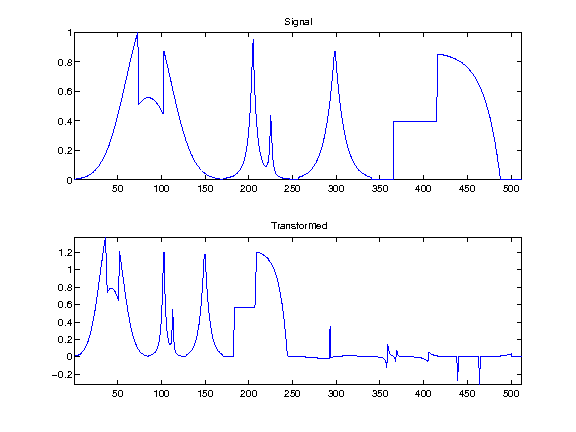The output of the forward transform is stored in the variable |fw|. At a given iteration indexed by a scale |j|, it will store in |fw(1:2^(j+1))| the variable $a_{j+1}$, and in the remaining entries the variable $d_{j+1},d_{j+2},\ldots,d_J$.

Initializes the algorithm at scale $j=J$.

In :
j = J;


Initialize |fw| to the full signal.

In :
fw = f;


At iteration indexed by $j$, select the sub-part of the signal containing $a_{j+1}$, and apply it the Haar operator.

In :
fw(1:2^(j+1)) = haar(fw(1:2^(j+1)));


Display the signal and its coarse coefficients.

In :
s = 400; t = 40;
clf;
subplot(2,1,1);
plot(f,'.-'); axis([s-t s+t 0 1]); title('Signal (zoom)');
subplot(2,1,2);
plot(fw(1:2^j),'.-'); axis([(s-t)/2 (s+t)/2 min(fw(1:2^j)) max(fw(1:2^j))]); title('Averages (zoom)');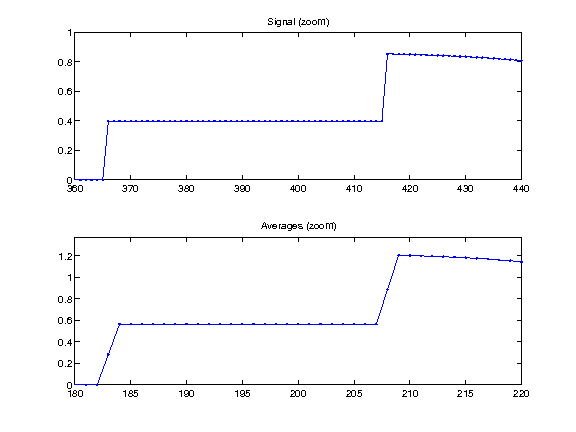Exercise 1

Implement a full wavelet Haar transform that extract iteratively wavelet coefficients, by repeating these steps. Take care of choosing the correct number of steps.

In :
exo1()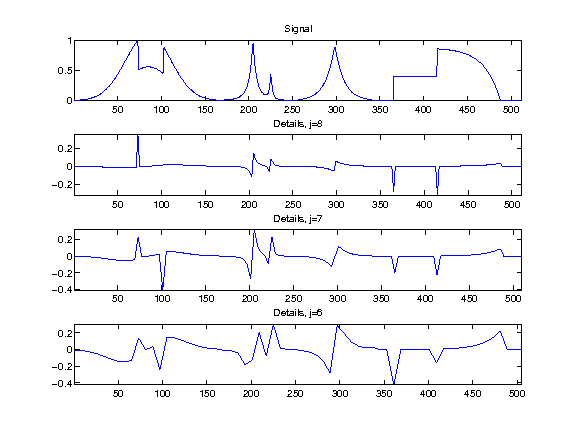In :
%% Insert your code here.


Check that the transform is orthogonal, which means that the energy of the coefficient is the same as the energy of the signal.

In :
fprintf('Should be 0: %.3f.\n', (norm(f)-norm(fw))/norm(f));

Should be 0: 0.000.


We display the whole set of coefficients |fw|, with red vertical separator between the scales. Can you recognize where are the low frequencies and the high frequencies?

In :
clf;
plot_wavelet(fw);
axis([1 N -2 2]);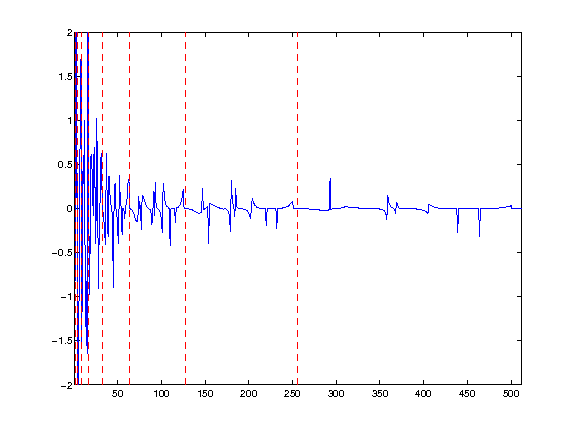## Backward Haar Transform¶

The backward transform computes a signal $f_1 = \Hh^*(h)$ from a set of coefficients $h = (d_j)_{j=0}^J \cup \{a_0\}$

If $h = \Hh(f)$ are the coefficients of a signal $f$, one recovers $f_1 = f$.

The inverse transform starts from $j=0$, and computes $a_{j+1}$ from the knowledge of $a_j,d_j$ as $$\forall k = 0,\ldots,2^j-1, \quad a_{j+1}[2k] = \frac{ a_j[k] + d_j[k] }{\sqrt{2}} a_{j+1}[2k+1] = \frac{ a_j[k] - d_j[k] }{\sqrt{2}}$$

One step of inverse transform

In :
ihaar = @(a,d)assign( zeros(2*length(a),1), ...
[1:2:2*length(a), 2:2:2*length(a)], [a+d; a-d]/sqrt(2) );


Initialize the signal to recover $f_1$ as the transformed coefficient, and select the smallest possible scale $j=0$.

In :
f1 = fw;
j = 0;


Apply one step of inverse transform.

In :
f1(1:2^(j+1)) = ihaar(f1(1:2^j), f1(2^j+1:2^(j+1)));


Exercise 2

Write the inverse wavelet transform that computes |f1| from the coefficients |fw|.

In :
exo2()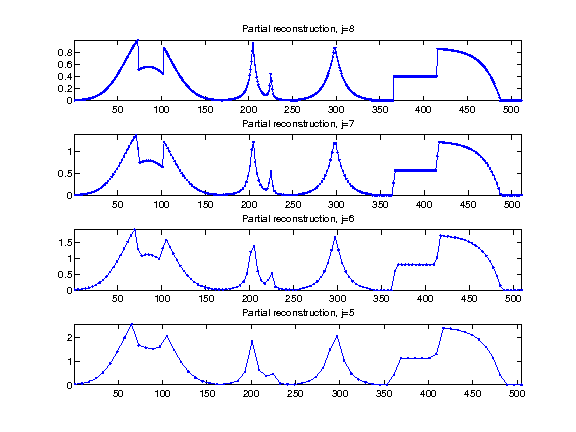In :
%% Insert your code here.


Check that we have correctly recovered the signal.

In :
fprintf('Should be 0: %.3f.\n', (norm(f-f1))/norm(f));

Should be 0: 0.000.


## Haar Linear Approximation¶

Linear approximation is obtained by setting to zeros the Haar coefficient coefficients above a certain scale $j$.

Cut-off scale.

In :
j = J-1;


Set to zero fine scale coefficients.

In :
fw1 = fw; fw1(2^j+1:end) = 0;


Computing the signal $f_1$ corresponding to these coefficients |fw1| computes the orthogonal projection on the space of signal that are constant on disjoint intervals of length $N/2^j$.

Exercise 3

Display the reconstructed signal obtained from |fw1|, for a decreasing cut-off scale $j$.

In :
exo3()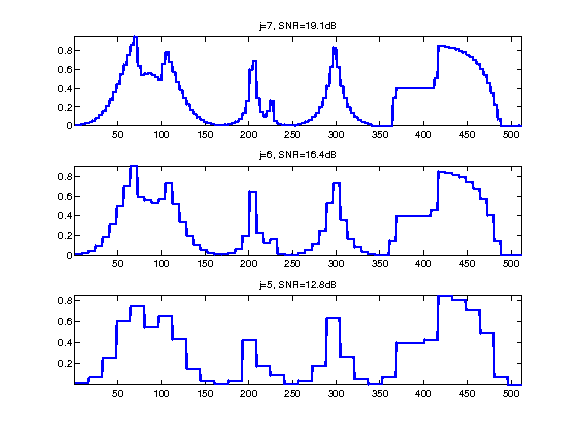In :
%% Insert your code here.


## Haar Non-linear Approximation¶

Non-linear approximation is obtained by thresholding low amplitude wavelet coefficients. It is defined as $$f_T = \Hh^* \circ S_T \circ \Hh(f)$$ where $S_T$ is the hard tresholding operator $$S_T(x)_i = \choice{ x_i \qifq \abs{x_i}>T, \\ 0 \quad \text{otherwise}. }.$$

In :
S = @(x,T) x .* (abs(x)>T);


Set the threshold value.

In :
T = .5;


Threshold the coefficients.

In :
fwT = S(fw,T);


Display the coefficients before and after thresholding.

In :
clf;
subplot(2,1,1);
plot_wavelet(fw); axis('tight'); title('Original coefficients');
subplot(2,1,2);
plot_wavelet(fwT); axis('tight'); title('Thresholded coefficients');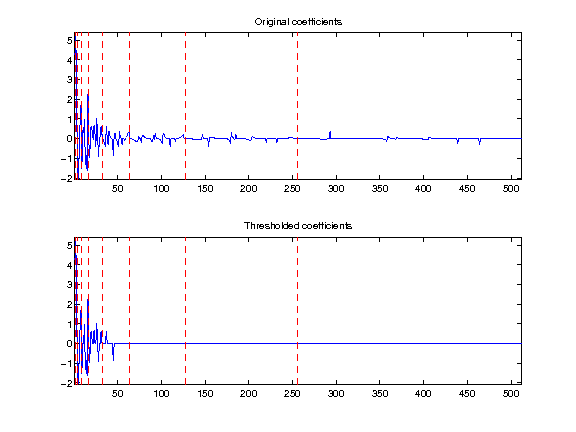Exercise 4

Find the threshold $T$ so that the number of remaining coefficients in |fwT| is a fixed number $m$. Use this threshold to compute |fwT| and then display the corresponding approximation $f_1$ of $f$. Try for an increasing number $m$ of coeffiients. ompute the threshold T

In :
exo4()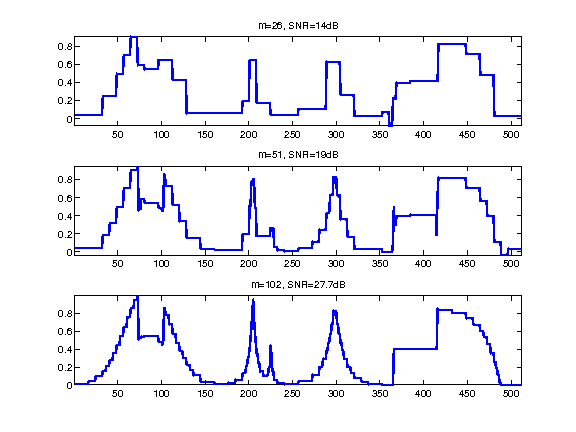In :
%% Insert your code here.


## The Shape of a Wavelet¶

A wavelet coefficient corresponds to an inner product of $f$ with a wavelet Haar atom $\psi_{j,k}$ $$d_j[k] = \dotp{f}{\psi_{j,k}}$$

The wavelet $\psi_{j,k}$ can be computed by applying the inverse wavelet transform to |fw| where |fw[m]=1| and |fw[s]=0| for $s \neq m$ where $m = 2^j+k$.

Exercise 5

Compute wavelets at several positions and scales.

In :
exo5()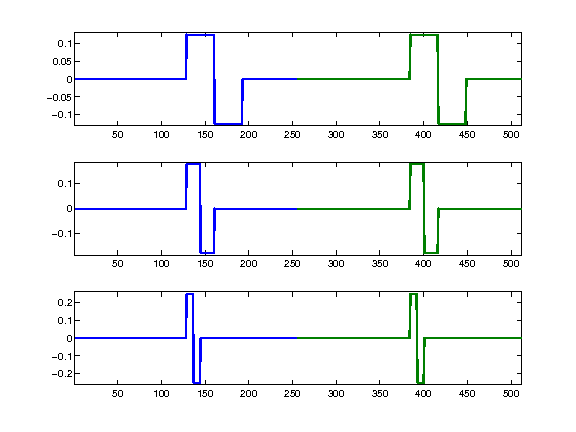In :
%% Insert your code here.